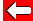# Averages: Mean

If we know the mean average of a set of numbers, we can use it to work out the total present.

e.g. Mr. Davis's class of 30 pupils have been raising money for charity. The average (mean) amount raised per pupils was £9.50. How much was raised in total?

Mean average = total of quantities / number of quantities

So the total = mean average × number of pupils
= £9.50 × 30
= £285·00

Didn't they do well?!Go back a pageMaths MenuGo to next page